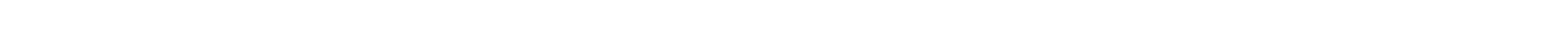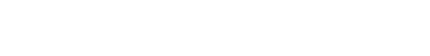## Machine Learning Tutorial

Machine learning is a subclass of Artificial Intelligence that gives the ability for the programs to learn without being explicitly programmed to do so. Before beginning to work on a machine learning problem, it is important to determine if it actually requires machine learning or not. It is also important to collect the right data, prepare the data in the right way and clean the data before providing it as input data to the learning model. Before this comes the environment set up: There are a wide variety of modules and frameworks that can be used to implement machine learning algorithms. Packages can be installed using the ‘pip’ command. Suppose we wish to install the scikit-learn package, we can do so using the below command on the command line. pip install scikit-learn The Machine Learning algorithms extract patterns from data and learn from them, like how humans learn based on experiences. Machine Learning algorithms can be classified into different types of learning based on the input and the type of input that is supplied. Machine Learning is widely used for applications such as data mining, computer vision, Natural Language Processing, Search engines, credit card fraud detection, speech and handwriting recognition, strategy games, robotics, and much more. It can be visualized with the help of the below image: Supervised learning It is one of the most popular learning methods, since it is easy to understand and relatively easier to implement ad get relevant outputs. Consider this example: How does a child learn? It is taught how to walk, run, talk, and it is made to understand the difference between walking and running. Supervised learning works in a similar way, there is human supervision involved in the form of features being labelled, feedback given to the data (whether it predicted correctly, and if not what the right prediction has to be) and so on. Once the algorithm has been fully trained on such data, it can predict outputs for never-before-seen inputs in-line with the data on which the model was trained with good accuracy. It is also understood as a task-oriented algorithm since it focuses on a single task and is trained on huge number of examples until it predicts output accurately. Semi-supervised learning It comes in between the supervised and unsupervised learning algorithms. It exists so as to bridge the gap that occurs due to usage of supervised and unsupervised algorithms. Supervised learning algorithms are expensive, meaning they need to be labelled (by a human). On the other hand, unsupervised learning algorithms might not be very accurate and might not be applicable in every field. In semi-supervised learning algorithm, the input data is a combination of labelled and unlabelled dataset. There is a small amount of labelled data and a comparatively large amount of unlabelled data. Similar kind of data is clustered into a single unit with the help of an unsupervised learning algorithm. The labelled data is used to label the unlabelled data further. Unsupervised learning This is the opposite of supervised learning, wherein no labelling is provided on the input data that is supplied to the unsupervised learning algorithm. The algorithm has to learn from the unlabelled data and perform operations over it on its own. Most of the times, real-world data is unstructured and unlabelled. Hence unsupervised algorithms need to be used. Otherwise humans interfere and label the input data so that it can be passed as input to a supervised learning algorithm. Consider this example: A set of images of horses in different angles, colours. No label is provided to the unsupervised learning algorithm indicating that all the images are that of horses. The algorithm itself learns from the images based on the features of these images, similarities, and differences. Reinforcement learning An algorithm that defines a reinforcement agent that decides what step has to be taken next so as to arrive at the result or find the optimal path. When no dataset is provided to a reinforcement learning algorithm, it learns from its surroundings and experiences. When an action is taken by the reinforcement algorithm, it is either awarded or punished (ways of awards and punishments differ based on the data available). If the algorithm is awarded, it moves in the same direction or on the same lines. On the other hand, if the algorithm is punished, it understands that it needs to find out a different way to arrive at the solution. How is it different from supervised learning algorithms? Supervised learning algorithm have an input and the expected output, whereas in reinforcement learning, the algorithm has to decide what action it needs to take next. Implementing the simplest machine learning algorithm, i.e. Linear regression, which is considered to be the ‘Hello World’ program in the field of machine learning: import numpy as np  import matplotlib.pyplot as plt  from sklearn.linear_model import LinearRegression  from sklearn.metrics import mean_squared_error, r2_score  #A random data set is generated  np.random.seed(0)  x = np.random.rand(100, 1)  y = -3.5 + 5.19* x + np.random.rand(100, 1)  #The model is initialized  regression_model = LinearRegression() The data is fit on the model, with the help of training regression_model.fit(x, y)  The output is predicted  y_predicted = regression_model.predict(x)  The model built is evaluated using mean squared error parameter rmse = mean_squared_error(y, y_predicted)  r2 = r2_score(y, y_predicted)  print("The slope value is: ", regression_model.coef_)  print("The intercept is: ", regression_model.intercept_)  print("The Root mean squared error is: ", rmse)  #The data is visualized usign the matplotlib library  plt.scatter(x, y, s=8)  plt.xlabel('X axis')  plt.ylabel('Y axis')  The values that are predicted plt.plot(x, y_predicted, color='g') plt.show() Output: The slope value is: [[5.12655106]]  The intercept is: [-2.94191998]  The Root mean squared error is: 0.07623324582875007 Conclusion In this post, we understood how the environment can be set up, the important of data preparation, cleaning, and the difference between training, testing and validation datasets.

# Introduction To Machine Learning using Python

Machine learning is a subclass of Artificial Intelligence that gives the ability for the programs to learn without being explicitly programmed to do so. Before beginning to work on a machine learning problem, it is important to determine if it actually requires machine learning or not. It is also important to collect the right data, prepare the data in the right way and clean the data before providing it as input data to the learning model.

### Before this comes the environment set up:

There are a wide variety of modules and frameworks that can be used to implement machine learning algorithms. Packages can be installed using the ‘pip’ command. Suppose we wish to install the scikit-learn package, we can do so using the below command on the command line.

pip install scikit-learn

The Machine Learning algorithms extract patterns from data and learn from them, like how humans learn based on experiences. Machine Learning algorithms can be classified into different types of learning based on the input and the type of input that is supplied. Machine Learning is widely used for applications such as data mining, computer vision, Natural Language Processing, Search engines, credit card fraud detection, speech and handwriting recognition, strategy games, robotics, and much more.

It can be visualized with the help of the below image:### Supervised learning

It is one of the most popular learning methods, since it is easy to understand and relatively easier to implement ad get relevant outputs.

Consider this example: How does a child learn? It is taught how to walk, run, talk, and it is made to understand the difference between walking and running.

Supervised learning works in a similar way, there is human supervision involved in the form of features being labelled, feedback given to the data (whether it predicted correctly, and if not what the right prediction has to be) and so on.

Once the algorithm has been fully trained on such data, it can predict outputs for never-before-seen inputs in-line with the data on which the model was trained with good accuracy. It is also understood as a task-oriented algorithm since it focuses on a single task and is trained on huge number of examples until it predicts output accurately.

### Semi-supervised learning

It comes in between the supervised and unsupervised learning algorithms.

It exists so as to bridge the gap that occurs due to usage of supervised and unsupervised algorithms.

Supervised learning algorithms are expensive, meaning they need to be labelled (by a human). On the other hand, unsupervised learning algorithms might not be very accurate and might not be applicable in every field.

In semi-supervised learning algorithm, the input data is a combination of labelled and unlabelled dataset. There is a small amount of labelled data and a comparatively large amount of unlabelled data. Similar kind of data is clustered into a single unit with the help of an unsupervised learning algorithm. The labelled data is used to label the unlabelled data further.

### Unsupervised learning

This is the opposite of supervised learning, wherein no labelling is provided on the input data that is supplied to the unsupervised learning algorithm. The algorithm has to learn from the unlabelled data and perform operations over it on its own. Most of the times, real-world data is unstructured and unlabelled. Hence unsupervised algorithms need to be used. Otherwise humans interfere and label the input data so that it can be passed as input to a supervised learning algorithm.

Consider this example: A set of images of horses in different angles, colours. No label is provided to the unsupervised learning algorithm indicating that all the images are that of horses. The algorithm itself learns from the images based on the features of these images, similarities, and differences.

### Reinforcement learning

An algorithm that defines a reinforcement agent that decides what step has to be taken next so as to arrive at the result or find the optimal path. When no dataset is provided to a reinforcement learning algorithm, it learns from its surroundings and experiences. When an action is taken by the reinforcement algorithm, it is either awarded or punished (ways of awards and punishments differ based on the data available). If the algorithm is awarded, it moves in the same direction or on the same lines. On the other hand, if the algorithm is punished, it understands that it needs to find out a different way to arrive at the solution.

How is it different from supervised learning algorithms?

Supervised learning algorithm have an input and the expected output, whereas in reinforcement learning, the algorithm has to decide what action it needs to take next.

Implementing the simplest machine learning algorithm, i.e. Linear regression, which is considered to be the ‘Hello World’ program in the field of machine learning:

import numpy as np
import matplotlib.pyplot as plt
from sklearn.linear_model import LinearRegression
from sklearn.metrics import mean_squared_error, r2_score
#A random data set is generated
np.random.seed(0)
x = np.random.rand(100, 1)
y = -3.5 + 5.19* x + np.random.rand(100, 1)
#The model is initialized
regression_model = LinearRegression()
The data is fit on the model, with the help of training regression_model.fit(x, y)
The output is predicted
y_predicted = regression_model.predict(x)
The model built is evaluated using mean squared error parameter rmse = mean_squared_error(y, y_predicted)
r2 = r2_score(y, y_predicted)
print("The slope value is: ", regression_model.coef_)
print("The intercept is: ", regression_model.intercept_)
print("The Root mean squared error is: ", rmse)
#The data is visualized usign the matplotlib library
plt.scatter(x, y, s=8)
plt.xlabel('X axis')
plt.ylabel('Y axis')
The values that are predicted plt.plot(x, y_predicted, color='g') plt.show() 

Output

The slope value is: [[5.12655106]]
The intercept is: [-2.94191998]
The Root mean squared error is: 0.07623324582875007 

#### Conclusion

In this post, we understood how the environment can be set up, the important of data preparation, cleaning, and the difference between training, testing and validation datasets.#### Vinu

After reading your article, I was amazed. I know that you explain it very well. And I hope that other readers will also experience how I feel after reading your article. Thanks for sharing.#### Johnson M

Good and informative article.#### Vinu

I enjoyed reading your articles. This is truly a great read for me. Keep up the good work!#### Vinu